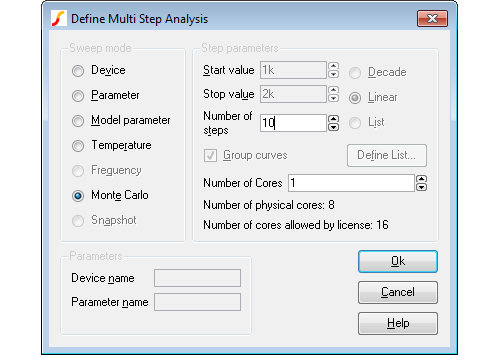Running Monte Carlo

In this topic:

Overview

There are actually two types of Monte Carlo analyses. These are:

1. Single step Monte Carlo sweep
2. Multi step Monte Carlo run.
1. above is applicable to AC, DC, Noise and Transfer Function analyses. 2. can be applied to the same analyses in addition to transient analysis.

An example of 1. can be seen in the figure in Monte Carlo. This was a run where the gain at a single frequency was calculated 1000 times with the Monte Carlo tolerances applied. This used AC analysis with the Monte Carlo sweep mode - one of the six modes available. Only a single curve is created hence the name single step.

An example of 2 is the example at the beginning of this chapter. Here a complete frequency sweep from 1kHz to 100kHz was repeated 100 times creating 100 curves.

Setting up a Single Step Monte Carlo Sweep

1. Select schematic menu Simulator > Choose Analysis.... Select the AC, DC, Noise or TF tab as required.
2. In the Sweep Parameters section, press the Define... button.
3. In the Sweep Mode section select Monte Carlo.
4. In the Parameters section enter the required value for the Number of points.
5. For AC, Noise and TF, you must also supply a value for Frequency.

Setting up a Multi Step Monte Carlo Run

1. Select schematic menu Simulator > Choose Analysis.... Select the AC, DC, Noise, Transient or TF tab as required.
2. Define the analysis as required.
3. In the Monte Carlo and Multi-step Analysis section, check the Enable multi-step box then press the Define... button. This will open:-4. In the Sweep mode section, select Monte Carlo.
5. In the Step Parameters section, enter the number of steps required.
6. You can set the Number of Cores to a number large than 1 if your system and license permit it. This will substantially speed up the run. For more information see Using Multiple Cores for Multi-step Analyses.

Running a Monte Carlo Analysis

Monte Carlo analyses are run in exactly the same way as other analyses. Press F9 or equivalent menu.

Log File

Multi-step Monte Carlo analyses generate a log file that documents the values of all toleranced parts. It will also record the seed value used for each step. To open the log file, select menu Monte Carlo > View Log File. If you specify more than one core for the run, this will open only the log file for the primary process. The log files for other processes may be found in folders multicore/pn where n is a number from 1 to the number of cores used less 1.

Setting the Seed Value

The random variations are created using a pseudo random number sequence. The sequence can be seeded such that it always produces the same sequence of numbers for a given seed. In Monte Carlo analysis, the random number generator is seeded with a new value at the start of each run and this seed value is displayed in the log file. It is also possible to fix the first seed that is used using the SEED option. This makes it possible to repeat a run. To do this, note the seed value of the run of interest from the log file then set the seed as follows:

1. Select schematic menu Simulator > Choose Analysis....
2. Select Options tab and enter the seed value in the Monte Carlo section.
The first run of each Monte Carlo analysis will use the same random values as the run from which you obtained the seed value in the log file. Note this assumes that only changes in values are made to the circuit. Any topology change will upset the sequence.

This technique is a convenient way of investigating a particular run that perhaps produced unexpected results. Obtain the seed used for that run, then repeat with the seed value but doing just a single run. You will then be able to probe around the circuit and plot the results for just that run.

Note that if you use more than one core for the run, the main log file will only show the data for the primary process. See Log File for more details.